Anzeige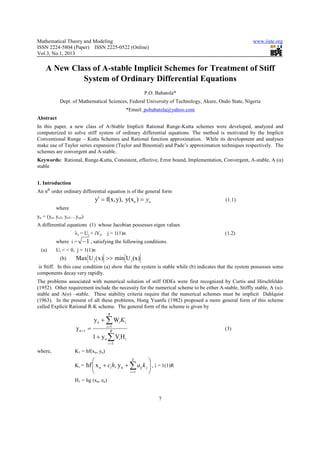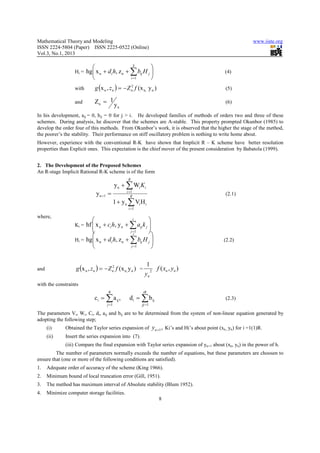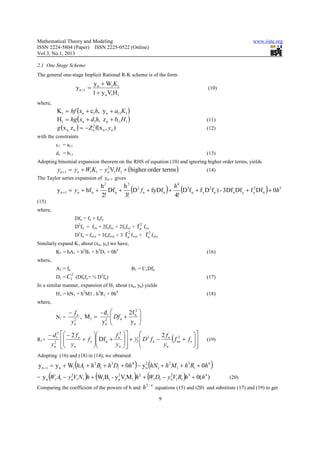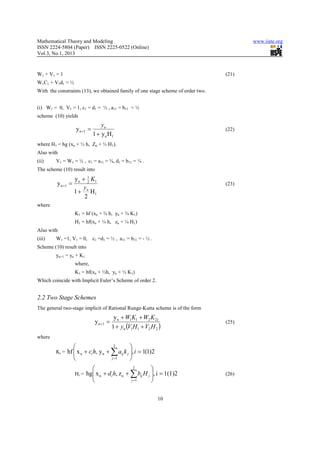Anzeige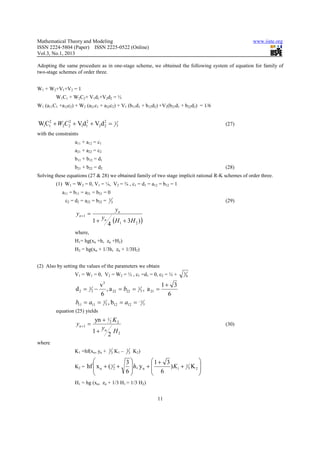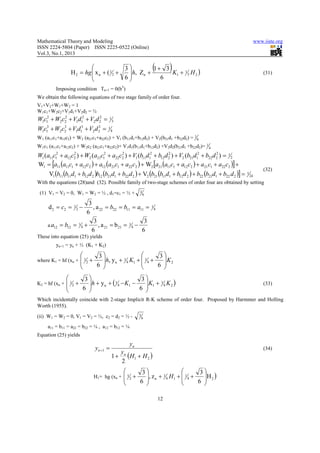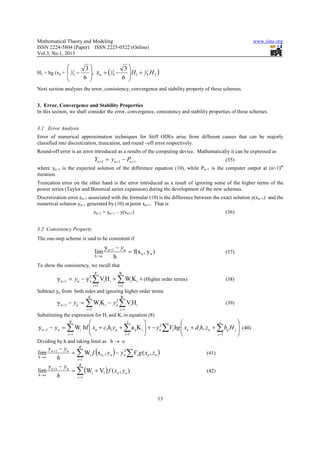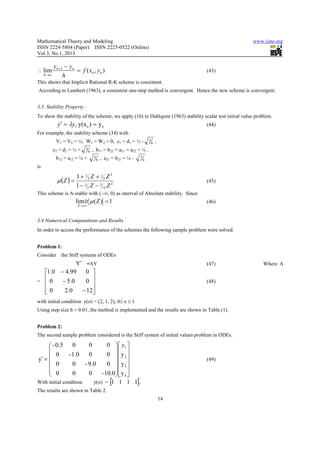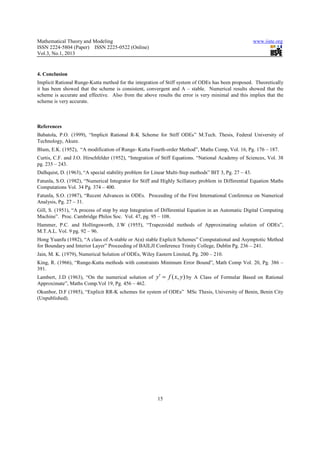Anzeige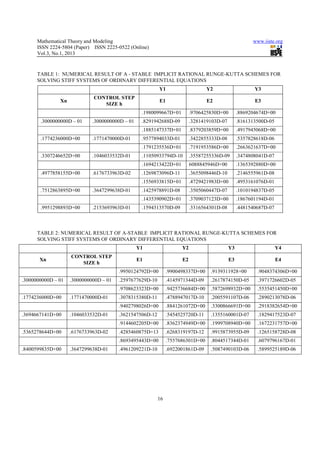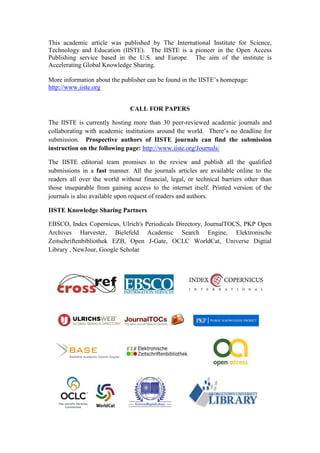Nächste SlideShare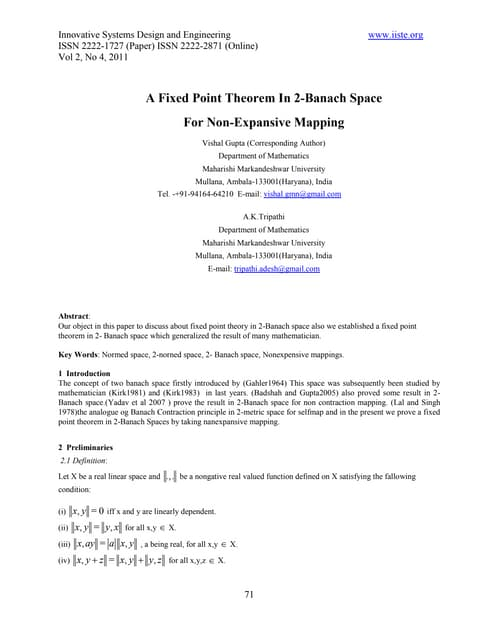6 adesh kumar tripathi -71-74
1 von 11
Anzeige

### A new class of a stable implicit schemes for treatment of stiff

1. Mathematical Theory and Modeling www.iiste.org ISSN 2224-5804 (Paper) ISSN 2225-0522 (Online) Vol.3, No.1, 2013 A New Class of A-stable Implicit Schemes for Treatment of Stiff System of Ordinary Differential Equations P.O. Babatola* Dept. of Mathematical Sciences, Federal University of Technology, Akure, Ondo State, Nigeria *Email: pobabatola@yahoo.com Abstract In this paper, a new class of A-Stable Implicit Rational Runge-Kutta schemes were developed, analyzed and computerized to solve stiff system of ordinary differential equations. The method is motivated by the Implicit Conventional Runge – Kutta Schemes and Rational function approximation. While its development and analyses make use of Taylor series expansion (Taylor and Binomial) and Pade’s approximation techniques respectively. The schemes are convergent and A-stable. Keywords: Rational, Runge-Kutta, Consistent, effective, Error bound, Implementation, Convergent, A-stable, A (α) stable 1. Introduction An nth order ordinary differential equation is of the general form y′ = f(x, y), y(xo ) = yo (1.1) where yo = (yo, yo2, yo3…yon) A differential equations (1) whose Jacobian possesses eigen values λj = Uj + iVj, j = 1(1)n (1.2) where i = − 1 , satisfying the following conditions. (a) Ui < < 0, j = 1(1)n (b) Max U j ( x ) >> min U j (x) is Stiff. In this case condition (a) show that the system is stable while (b) indicates that the system possesses some components decay very rapidly. The problems associated with numerical solution of stiff ODEs were first recognized by Curtis and Hirschfelder (1952). Other requirement include the necessity for the numerical scheme to be either A-stable, Stiffly stable, A (α)- stable and A(o) –stable. These stability criteria require that the numerical schemes must be implicit Dahlquist (1963). In the present of all these problems, Hong Yuanfu (1982) proposed a more general form of this scheme called Explicit Rational R-K scheme. The general form of the scheme is given by R y n + ∑ Wi K i y n +1 = i =1 R (3) 1 + y n ∑ Vi H i i =1 where, K1 = hf(xn, yn)  S  Ki = hf  x n + ci h, y n + ∑ aij k j  , i = 1(1)R  i =1  H1 = hg (xn, zn) 7
2. Mathematical Theory and Modeling www.iiste.org ISSN 2224-5804 (Paper) ISSN 2225-0522 (Online) Vol.3, No.1, 2013  S  Hi = hg x n + di h, z n + ∑ bij H j  (4)  i =1  with g (x n , zn ) = − Z n f (x n, y n ) 2 (5) and Zn = 1 (6) yn In his development, aij = 0, bij = 0 for j > i. He developed families of methods of orders two and three of these schemes. During analysis, he discover that the schemes are A-stable. This property prompted Okunbor (1985) to develop the order four of this methods. From Okunbor’s work, it is observed that the higher the stage of the method, the poorer’s the stability. Their performance on stiff oscillatory problem is nothing to write home about. However, experience with the conventional R-K have shown that Implicit R – K scheme have better resolution properties than Explicit ones. This expectation is the chief mover of the present consideration by Babatola (1999). 2. The Development of the Proposed Schemes An R-stage Implicit Rational R-K scheme is of the form R y n + ∑ Wi K i y n +1 = i =1 R (2.1) 1 + y n ∑ Vi H i i =1 where,  i  Ki = hf  x n + ci h, y n + ∑ aij k j     j =1   i  Hi = hg  x n + di h, z n + ∑ bij H j  (2.2)    j =1  1 and g (x n , zn ) = − Z n2 f (x n, y n ) = 2 f ( xn , yn ) yn with the constraints R iR ci = ∑ a ij , d i = ∑ b ij (2.3) j =1 jj =1 The parameters Vi, Wi, Ci, di, aij and bij are to be determined from the system of non-linear equation generated by adopting the following step; (i) Obtained the Taylor series expansion of yn +1 , Ki’s and Hi’s about point (xn,, yn) for i =1(1)R. (ii) Insert the series expansion into (7). (iii) Compare the final expansion with Taylor series expansion of yn+1 about (xn, yn) in the power of h. The number of parameters normally exceeds the number of equations, but these parameters are choosen to ensure that (one or more of the following conditions are satisfied). 1. Adequate order of accuracy of the scheme (King 1966). 2. Minimum bound of local truncation error (Gill, 1951). 3. The method has maximum interval of Absolute stability (Blum 1952). 4. Minimize computer storage facilities. 8
3. Mathematical Theory and Modeling www.iiste.org ISSN 2224-5804 (Paper) ISSN 2225-0522 (Online) Vol.3, No.1, 2013 2.1 One Stage Scheme The general one-stage Implicit Rational R-K scheme is of the form y n + W1K1 y n +1 = (10) 1 + y n V1H1 where, K1 = hf ( xn + c1h, y n + a11K1 ) H1 = hg ( xn + d1h, z n + b11H1 ) (11) g (x n z n ) = − Z n2f(x n , yn ) (12) with the constraints c1 = a11 d1 = b11 (13) Adopting binomial expansion theorem on the RHS of equation (10) and ignoring higher order terms, yields yn +1 = yn + W1K1 − yn V1H1 + (higher order terms ) 2 (14) The Taylor series expansion of yn+1 gives h2 h3 2 h4 3 y n +1 = yn + hf n + 2! Df n + 3! ( D f n + fyDfn + 4! ) ( D f n + f y D 2f n ) - 3Df n Df y + f y2 Df n + 0h5 ) (15) where, Dfn = fx + fnfy D2fn = fxx + 2fnfxy + 2fnfxy + f n2 fyy 2 3 D3fn = fxxx + 3fnfxxy + 3 f n fxyy + f n fyyy Similarly expand K1 about (xn, yn) we have, K1 = hA1 + h2B1 + h3D1 + 0h4 (16) where, A 1 = fn B1 = C1Dfn 2 2 D1 = C (Dfnfy+ ½ D fn) 1 (17) In a similar manner, expansion of H1 about (xn, yn) yields H1 = hN1 + h2M1 + h3R1 + 0h4 (18) where, − fn - d1  2f 2  N1 = , M1 = 2   Df n + n  2 yn yn  yn   − d12  − 2 f n  f 2    R1 = 2      2f + f y  Df n + n  + 1 2  D 2 f n − n f nn + f x  2  ( (19) yn  yn   yn    yn  Adopting (16) and (18) in (14), we obtained ( ) ( y n +1 = y n + W1 hA1 + h 2 B1 + h3 D1 + 0h 4 − y 2 hN1 + h 2 M1 + h3 R1 + 0h 4 n ) = y (W A − y V N )h + (W B - y V M )h + (W D − y V R )h + 0(h 4 ) 2 2 2 2 3 n 1 1 n 1 1 1 1 n 1 1 1 1 n 1 1 (20) 2/n Comparing the coefficient of the powers of h and h equations (15) and (20) and substitute (17) and (19) to get 9
4. Mathematical Theory and Modeling www.iiste.org ISSN 2224-5804 (Paper) ISSN 2225-0522 (Online) Vol.3, No.1, 2013 W1 + V1 = 1 (21) W1C1 + V1d1 = ½ With the constraints (13), we obtained family of one stage scheme of order two. (i) W1 = 0, V1 = 1, c1 = d1 = ½ , a11 = b11 = ½ scheme (10) yields yn y n +1 = (22) 1 + y n H1 where H1 = hg (xn + ½ h, Zn + ½ H1). Also with (ii) V1 = W1 = ½ , c1 = a11 = ¾, d1 = b11 = ¼ . The scheme (10) result into y n + 1 K1 y n +1 = 2 (23) yn 1 + H1 2 where K1 = hf (xn + ¾ h, yn + ¾ K1) H1 = hf(xn + ¼ h, zn + ¼ H1) Also with (iii) W1 =1, V1 = 0, c1 =d1 = ½ , a11 = b11 = - ½ . Scheme (10) result into yn+1 = yn + K1 where, K1 = hf(xn + ½h, yn + ½ K1) Which coincide with Implicit Euler’s Scheme of order 2. 2.2 Two Stage Schemes The general two-stage implicit of Rational Runge-Kutta scheme is of the form y n + W1K1 + W2 K 21 y n +1 = (25) 1 + yn (V1H1 + V2 H 2 ) where  2  Ki = hf  x n + ci h, y n + ∑ aij k j , i = 1(1)2    j =1   2  Hi = hg x n + d i h, z n + ∑ bij H j , i = 1(1)2   (26)  j =1  10
5. Mathematical Theory and Modeling www.iiste.org ISSN 2224-5804 (Paper) ISSN 2225-0522 (Online) Vol.3, No.1, 2013 Adopting the same procedure as in one-stage scheme, we obtained the following system of equation for family of two-stage schemes of order three. W1 + W2+V1+V2 = 1 W1C1 + W2C2+ V1d1+V2d2 = ½ W1 (a11C1 +a12c2) + W2 (a21c1 + a22c2) + V1 (b11d1 + b12d2) +V2(b21d1 + b22d2) = 1/6 W1C1 + W2C2 + V1d1 + V2d 2 = 2 2 2 2 1 3 (27) with the constraints a11 + a12 = c1 a21 + a22 = c2 b11 + b12 = d1 b21 + b22 = d2 (28) Solving these equations (27 & 28) we obtained family of two stage implicit rational R-K schemes of order three. (1) W1 = W2 = 0, V1 = ¼, V2 = ¾ , c1 = d1 = a12 = b12 = 1 a11 = b11 = a21 = b21 = 0 c2 = d2 = a22 = b22 = 1 (29) 3 yn yn +1 = 1+ yn 4 (H 1 + 3 H 2 ) ) where, H1= hg(xn +h, zn +H2) H2 = hg(xn + 1/3h, zn + 1/3H2) (2) Also by setting the values of the parameters we obtain V1 = W1 = 0, V2 = W2 = ½ , c1 =d1 = 0, c2 = ½ + 3 6 v 3 1+ 3 d2 = 1 2 − , a 22 = b22 = 13 , a 21 = 6 6 b11 = a11 = 3 12 = a12 = 3 1 ,b −1 equation (25) yields yn + 1 2 K 2 yn +1 = (30) y 1+ n H2 2 where K1 =hf(xn, yn + 1 K1 – 1 K2) 3 3  3 1+ 3  K2 = hf  x n + ( 1 2 +  h, y n +  ) K1 + 13 K 2   6   6    H1 = hg (xn, zn + 1/3 H1 = 1/3 H2) 11
6. Mathematical Theory and Modeling www.iiste.org ISSN 2224-5804 (Paper) ISSN 2225-0522 (Online) Vol.3, No.1, 2013  H 2 = hg  x n + ( 1 2 + 3 h, Zn + 1+ 3 ( K1 + 1 3 H 2 ) ) (31)   6 6  Imposing condition Tn+1 = 0(h5) We obtain the following equations of two stage family of order four. V1+V2+W1+W2 = 1 W1c1+W2c2+V1d1+V2d2 = ½ W1c12 + W2c2 + V1d12 + V2 d 22 = 2 1 3 W c +W c +V d +V d = 3 1 1 3 2 2 3 1 1 3 2 2 1 4 W1 (a11c1+a12c2) + W2 (a21c1+a22c2) + V1 (b11d1+b12d2) + V2(b21d1 +b22d2) = 1 6 W1c1 (a11c1+a12c2) + W2c2 (a21c1+a22c2)+ V1d1(b11d1+b12d2) +V2d2(b21d1 +b22d2)= 1 4 W1 (a11c12 + a12c2 ) + W2 (a21c12 + a22c2 ) + V1 (b11d12 + b12d 2 ) + V2 (b21d12 + b22d 2 ) = 1 2 2 2 2 2 W1 = [a11 (a11c1 + a12c2 ) + a12 (a21c1 + a22c2 ) + W2 [a21 (a11c1 + a12c2 ) + a21 c1 + a22c2 )] + (32) V1 (b11 (b11d1 + b12 d 2 )b12 (b21d1 + b22 d 2 ) + V2 (b21 (b11d1 + b12 d 2 ) + b22 (b21d1 + b22 d 2 )] = 1 24 With the equations (28)and (32). Possible family of two-stage schemes of order four are obtained by setting (1) V1 = V2 = 0, W1 = W2 = ½ , d1=c1 = ½ + 3 6 3 d 2 = c2 = 1 2 − , a 22 = b22 = b11 = a11 = 1 4 6 3 3 a a12 = b12 = 1 4 + , a 21 = b 21 = 1 4 − 6 6 These into equation (25) yields yn+1 = yn + ½ (K1 + K2)  3  3 where K1 = hf (xn +  12 + h, y n + 1 4 K1 +  1 4 + K2  6   6       3 3  2 + 6 h + y n + ( 4 − K1 − 6  K1 + 4 K 2 ) K2 = hf (xn + 1  1  1 (33)    Which incidentally coincide with 2-stage Implicit R-K scheme of order four. Proposed by Harmmer and Holling Worth (1955). (ii) W1 = W2 = 0, V1 = V2 = ½, c2 = d2 = ½ - 3 6 a11 = b11 = a22 = b22 = ¼ , a12 = b12 = ¼ Equation (25) yields yn yn +1 = (34) y 1 + n ( H1 + H 2 ) 2  3  3 H1= hg (xn +  12 + , z n + 1 4 H1 +  1 4 + H 2 )  6   6      12
7. Mathematical Theory and Modeling www.iiste.org ISSN 2224-5804 (Paper) ISSN 2225-0522 (Online) Vol.3, No.1, 2013  3 3  2 − 6 , z n + ( 4 − 6  H1 + 4 H 2 ) H3 = hg (xn + 1  1  1    Next section analyses the error, consistency, convergence and stability property of these schemes. 3. Error, Convergence and Stability Properties In this section, we shall consider the error, convergence, consistency and stability properties of these schemes. 3.1 Error Analysis Error of numerical approximation techniques for Stiff ODEs arise from different causes that can be majorly classified into discretization, truncation, and round –off error respectively. Round-off error is an error introduced as a results of the computing device. Mathematically it can be expressed as Yn +1 = yn +1 − Pn +1 (35) where yn+1 is the expected solution of the difference equation (10), while Pn+1 is the computer output at (n+1)th iteration. Truncation error on the other hand is the error introduced as a result of ignoring some of the higher terms of the power series (Taylor and Binomial series expansion) during the development of the new schemes. Discretization error en+1 associated with the formular (10) is the difference between the exact solution y(xn+1) and the numerical solution yn+1 generated by (10) at point xn+1. That is en+1 = yn+1 – y(xn+1) (36) 3.2 Consistency Property The one-step scheme is said to be consistent if y n +1 − yn lim = f(x n , y n ) (37) h →o h To show the consistency, we recall that R R y n +1 = yn − y 2 ∑ Vi H i + ∑ Wi K i + (Higher order terms) n (38) i =1 i =1 Subtract yn from both sides and ignoring higher order terms R R y n +1 − yn = ∑ Wi K i − yn ∑ Vi H i 2 (39) i =1 i =1 Substituting the expression for Hi and Ki in equation (8) R  j   i  y n +1 − yn = ∑ Wi hf   xn + c1h1 yn + ∑ a ij K j  + − yn ∑Vi hg  xn + d i h, zn + ∑ bij H j  (40)  2   i =1  i =1   j =1  Dividing by h and taking limit as h → o yn +1 − yn R lim = ∑ W1 f (x n, , yn ) − yn ∑Vi g ( xn , zn ) 2 (41) h→o h i =1 y − yn R lim n +1 = ∑ (W1 + V1 ) f ( xn , yn ) (42) h →o h i =1 13
8. Mathematical Theory and Modeling www.iiste.org ISSN 2224-5804 (Paper) ISSN 2225-0522 (Online) Vol.3, No.1, 2013 yn +1 − yn ∴ lim = f ( xn , yn ) (43) h →o h This shows that Implicit Rational R-K scheme is consistent. According to Lambert (1963), a consistent one-step method is convergent. Hence the new scheme is convergent. 3.3. Stability Property To show the stability of the scheme, we apply (10) to Dahlquist (1963) stability scalar test initial value problem. y′ = λy, y(xo ) = yo (44) For example, the stability scheme (34) with V1 = V2 = ½, W1 = W2 = 0, c1 = d1 = ½ - 3 , 6 c2 = d2 = ½ + 3 , b11 = b22 = a11 = a22 = ¼ . 6 b12 = a12 = ¼ + 3 , a21 = b21 = ¼ - 3 6 6 is 1 + 12 Z + 12 Z 2 µ (Z ) = (45) 1 − 12 Z − 512 Z 2 This scheme is A-stable with ( -∞, 0) as interval of Absolute stability. Since lim it µ ( Z ) < 1 (46) Z →∞ 3.4 Numerical Computations and Results In order to access the performance of the schemes the following sample problem were solved. Problem 1: Consider the Stiff systems of ODEs Y′ =AY (47) Where A 1.0 − 4.99 0  = 0 − 5.0 0  (48)   0  2.0 − 12  with initial condition y(o) = (2, 1, 2), 0≤ x ≤ 1 Using step size h = 0.01, the method is implemented and the results are shown in Table (1). Problem 2: The second sample problem considered is the Stiff system of initial values problem in ODEs.  - 0.5 0 0 0   y1     0 - 1.0 0 0  y2    y′ =  (49) 0 0 - 9.0 0  y3      0 0 0 - 10.0   y 4    With initial condition y(o) = [1 1 1 1] , The results are shown in Table 2. 14
9. Mathematical Theory and Modeling www.iiste.org ISSN 2224-5804 (Paper) ISSN 2225-0522 (Online) Vol.3, No.1, 2013 4. Conclusion Implicit Rational Runge-Kutta method for the integration of Stiff system of ODEs has been proposed. Theoretically it has been showed that the scheme is consistent, convergent and A – stable. Numerical results showed that the scheme is accurate and effective. Also from the above results the error is very minimal and this implies that the scheme is very accurate. References Babatola, P.O. (1999), “Implicit Rational R-K Scheme for Stiff ODEs” M.Tech. Thesis, Federal University of Technology, Akure. Blum, E.K. (1952), “A modification of Runge- Kutta Fourth-order Method”, Maths Comp, Vol. 16, Pg. 176 – 187. Curtis, C.F. and J.O. Hirschfelder (1952), “Integration of Stiff Equations. “National Academy of Sciences, Vol. 38 pg. 235 – 243. Dalhquist, D. (1963), “A special stability problem for Linear Multi-Step methods” BIT 3, Pg. 27 – 43. Fatunla, S.O. (1982), “Numerical Integrator for Stiff and Highly Scillatory problem in Differential Equation Maths Computations Vol. 34 Pg. 374 – 400. Fatunla, S.O. (1987), “Recent Advances in ODEs. Proceeding of the First International Conference on Numerical Analysis, Pg. 27 – 31. Gill, S. (1951), “A process of step by step Integration of Differential Equation in an Automatic Digital Computing Machine”. Proc. Cambridge Philos Soc. Vol. 47, pg. 95 – 108. Hammer, P.C. and Hollingsworth, J.W (1955), “Trapezoidal methods of Approximating solution of ODEs”, M.T.A.L. Vol. 9 pg. 92 – 96. Hong Yuanfu (1982), “A class of A-stable or A(α) stable Explicit Schemes” Computational and Asymptotic Method for Boundary and Interior Layer” Proceeding of BAILJI Conference Trinity College, Dublin Pg. 236 – 241. Jain, M. K. (1979), Numerical Solution of ODEs, Wiley Eastern Limited, Pg. 200 – 210. King, R. (1966), “Runge-Kutta methods with constraints Minimum Error Bound”, Math Comp Vol. 20, Pg. 386 – 391. Lambert, J.D (1963), “On the numerical solution of y′ = f ( x, y) by A Class of Formular Based on Rational Approximate”, Maths Comp.Vol 19, Pg. 456 – 462. Okunbor, D.F (1985), “Explicit RR-K schemes for system of ODEs” MSc Thesis, University of Benin, Benin City (Unpublished). 15
10. Mathematical Theory and Modeling www.iiste.org ISSN 2224-5804 (Paper) ISSN 2225-0522 (Online) Vol.3, No.1, 2013 TABLE 1: NUMERICAL RESULT OF A - STABLE IMPLICIT RATIONAL RUNGE-KUTTA SCHEMES FOR SOLVING STIFF SYSTEMS OF ORDINARY DIFFERENTIAL EQUATIONS Y1 Y2 Y3 CONTROL STEP Xn E1 E2 E3 SIZE h .1980099667D+01 .9706425830D+00 .8869204674D+00 .3000000000D – 01 .3000000000D – 01 .8291942688D-09 .3281419103D-07 .8161313500D-05 .1885147337D+01 .8379203859D+00 .4917945068D+00 .1774236000D+00 .1771470000D-01 .9577894033D-01 .3422855333D-08 .5357828618D-06 .1791235536D+01 .7191953586D+00 .2663621637D+00 .3307246652D+00 .1046033532D-01 .11050933794D-10 .35587255336D-09 .3474808041D-07 .1694213422D+01 6088845946D+00 .1365392880D+00 .4977858155D+00 .6176733963D-02 .1269873096D-11 .3655098446D-10 .2146555961D-08 .1556933815D+01 .4729421983D+00 .4953161076D-01 .7512863895D+00 .3647299638D-01 .1425978891D-08 .3505060447D-07 .1010194837D-05 .1435390902D+01 .3709037123D+00 .1867601194D-01 .9951298893D+00 .2153693963D-01 .1594313570D-09 .3316564301D-08 .4481540687D-07 TABLE 2: NUMERICAL RESULT OF A-STABLE IMPLICIT RATIONAL RUNGE-KUTTA SCHEMES FOR SOLVING STIFF SYSTEMS OF ORDINARY DIFFERENTIAL EQUATIONS Y1 Y2 Y3 Y4 CONTROL STEP Xn E1 E2 E3 E4 SIZE h .9950124792D+00 .9900498337D+00 .9139311928+00 .9048374306D+00 .3000000000D – 01 .3000000000D – 01 .2597677629D-10 .4145971344D-09 .2617874150D-05 .3971726602D-05 .9708623323D+00 .9425736684D+00 .5872698932D+00 .5535451450D+00 .1774236000D+00 .1771470000D-01 .3078315380D-11 .4788947017D-10 .2005591107D-06 .2890213078D-06 .9402798026D+00 .8841261072D+00 .3300866691D+00 .2918382654D+00 .3694667141D+00 .1046033532D-01 .3621547506D-12 .5454525720D-11 .1355160001D-07 .1829417523D-07 .9144602205D+00 .8362374949D+00 .1999708940D+00 .1672231757D+00 .5365278644D+00 .6176733963D-02 .4285460875D+13 .6268319197D-12 .9915873955D-09 .1265158728D-08 .8693495443D+00 .7557686301D+00 .8044517344D-01 .6079796167D-01 .8400599835D+00 .3647299638D-01 .4961209221D-10 .6922001861D-09 .5087490103D-06 .5899525189D-06 16